# Optimisation random graph presentation

5 May 2017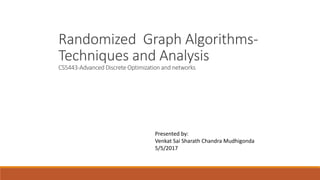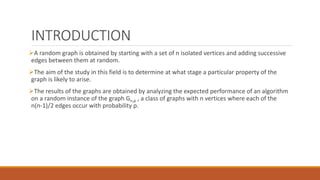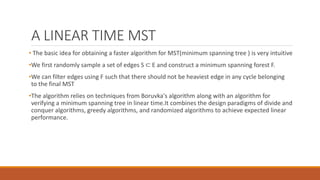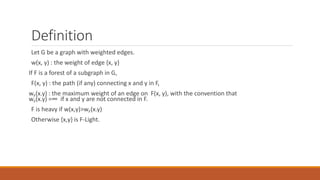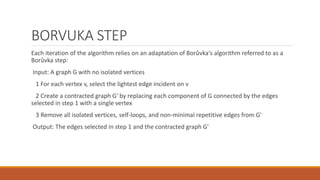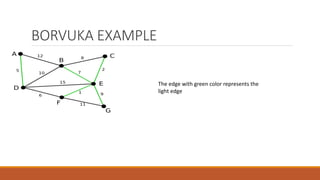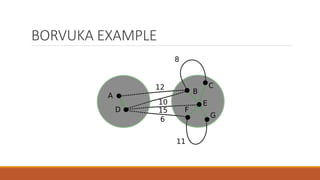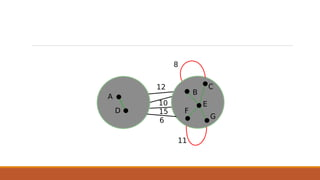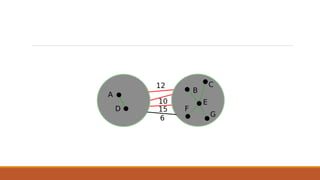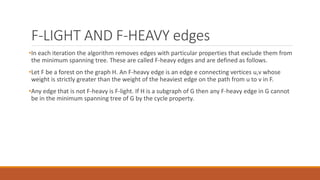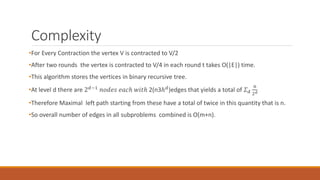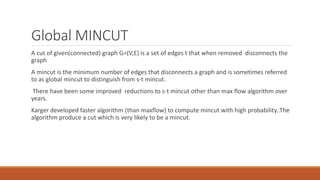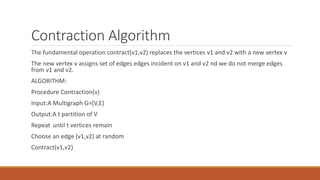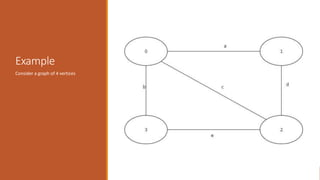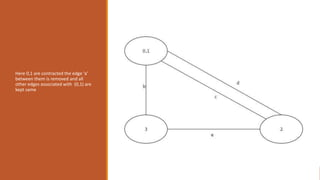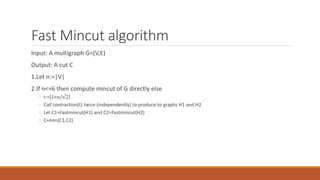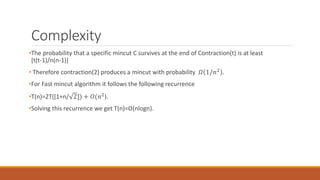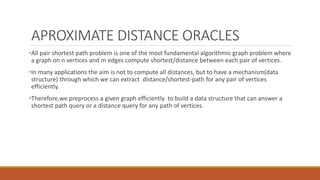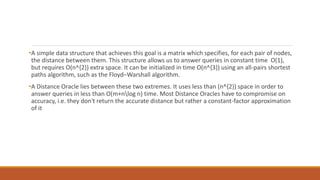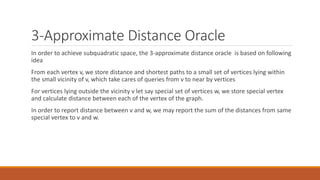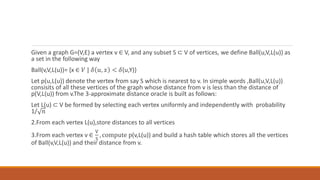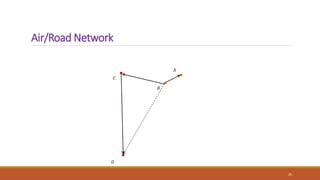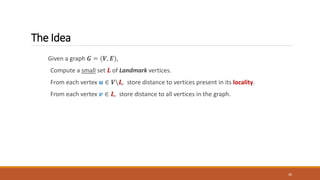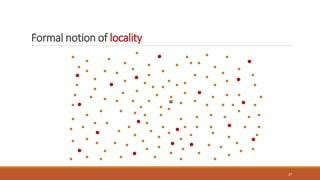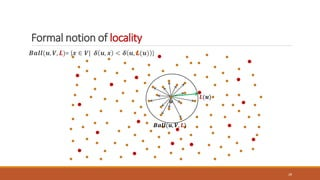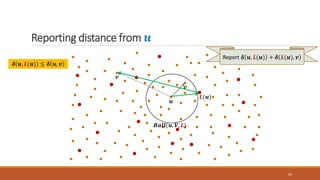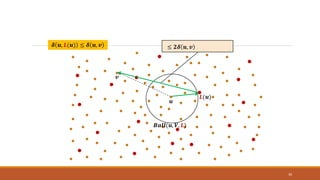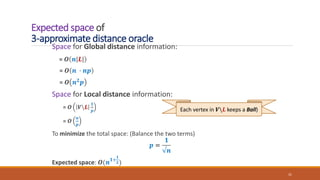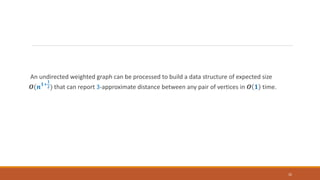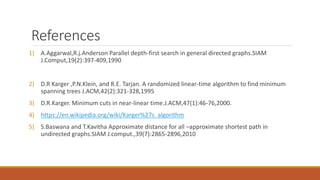1 sur 33

### Optimisation random graph presentation

• 1. Randomized Graph Algorithms- Techniques and Analysis CS5443-Advanced Discrete Optimization and networks Presented by: Venkat Sai Sharath Chandra Mudhigonda 5/5/2017
• 3. INTRODUCTION A random graph is obtained by starting with a set of n isolated vertices and adding successive edges between them at random. The aim of the study in this field is to determine at what stage a particular property of the graph is likely to arise. The results of the graphs are obtained by analyzing the expected performance of an algorithm on a random instance of the graph Gn,p , a class of graphs with n vertices where each of the n(n-1)/2 edges occur with probability p.
• 4. A LINEAR TIME MST • The basic idea for obtaining a faster algorithm for MST(minimum spanning tree ) is very intuitive •We first randomly sample a set of edges S ⊂ E and construct a minimum spanning forest F. •We can filter edges using F such that there should not be heaviest edge in any cycle belonging to the final MST •The algorithm relies on techniques from Boruvka's algorithm along with an algorithm for verifying a minimum spanning tree in linear time.It combines the design paradigms of divide and conquer algorithms, greedy algorithms, and randomized algorithms to achieve expected linear performance.
• 5. Definition Let G be a graph with weighted edges. w(x, y) : the weight of edge {x, y} If F is a forest of a subgraph in G, F(x, y) : the path (if any) connecting x and y in F, wF(x.y) : the maximum weight of an edge on F(x, y), with the convention that wF(x.y) =∞ if x and y are not connected in F. F is heavy if w(x,y)>wF(x.y) Otherwise {x,y} is F-Light.
• 6. To obtain a linear MST we need to follow two steps: 1. Boruvka Step 2. F-heavy and F-light edges
• 7. BORVUKA STEP Each iteration of the algorithm relies on an adaptation of Borůvka's algorithm referred to as a Borůvka step: Input: A graph G with no isolated vertices 1 For each vertex v, select the lightest edge incident on v 2 Create a contracted graph G' by replacing each component of G connected by the edges selected in step 1 with a single vertex 3 Remove all isolated vertices, self-loops, and non-minimal repetitive edges from G' Output: The edges selected in step 1 and the contracted graph G'
• 8. BORVUKA EXAMPLE The edge with green color represents the light edge
• 12. F-LIGHT AND F-HEAVY edges •In each iteration the algorithm removes edges with particular properties that exclude them from the minimum spanning tree. These are called F-heavy edges and are defined as follows. •Let F be a forest on the graph H. An F-heavy edge is an edge e connecting vertices u,v whose weight is strictly greater than the weight of the heaviest edge on the path from u to v in F. •Any edge that is not F-heavy is F-light. If H is a subgraph of G then any F-heavy edge in G cannot be in the minimum spanning tree of G by the cycle property.
• 13. Complexity •For Every Contraction the vertex V is contracted to V/2 •After two rounds the vertex is contracted to V/4 in each round t takes O(|E|) time. •This algorithm stores the vertices in binary recursive tree. •At level d there are 2 𝑑−1 𝑛𝑜𝑑𝑒𝑠 𝑒𝑎𝑐ℎ 𝑤𝑖𝑡ℎ 2(n3ℎ 𝑑)edges that yields a total of 𝛴 𝑑 n 2 𝑑 •Therefore Maximal left path starting from these have a total of twice in this quantity that is n. •So overall number of edges in all subproblems combined is O(m+n).
• 14. Global MINCUT A cut of given(connected) graph G=(V,E) is a set of edges t that when removed disconnects the graph A mincut is the minimum number of edges that disconnects a graph and is sometimes referred to as global mincut to distinguish from s-t mincut. There have been some improved reductions to s-t mincut other than max flow algorithm over years. Karger developed faster algorithm (than maxflow) to compute mincut with high probability..The algorithm produce a cut which is very likely to be a mincut.
• 15. Contraction Algorithm The fundamental operation contract(v1,v2) replaces the vertices v1 and v2 with a new vertex v The new vertex v assigns set of edges edges incident on v1 and v2 nd we do not merge edges from v1 and v2. ALGORITHM: Procedure Contraction(v) Input:A Multigraph G=(V,E) Output:A t partition of V Repeat until t vertices remain Choose an edge (v1,v2) at random Contract(v1,v2)
• 16. Example Consider a graph of 4 vertices
• 17. Here 0,1 are contracted the edge ‘a’ between them is removed and all other edges associated with (0,1) are kept same
• 19. Fast Mincut algorithm Input: A multigraph G=(V,E) Output: A cut C 1.Let n:=|V| 2.If n<=6 then compute mincut of G directly else ◦ t:=[1+n/√2] ◦ Call contraction(t) twice (independently) to produce to graphs H1 and H2 ◦ Let C1=Fastmincut(H1) and C2=Fastmincut(H2) ◦ C=min{C1,C2}
• 20. Complexity •The probability that a specific mincut C survives at the end of Contraction(t) is at least [t(t-1)/n(n-1)] • Therefore contraction(2) produces a mincut with probability 𝛺 1/𝑛2 . •For Fast mincut algorithm it follows the following recurrence •T(n)=2T([1+n/ 2]) + 𝑂(𝑛2 ). •Solving this recurrence we get T(n)=O(nlogn).
• 21. APROXIMATE DISTANCE ORACLES •All pair shortest path problem is one of the most fundamental algorithmic graph problem where a graph on n vertices and m edges compute shortest/distance between each pair of vertices. •In many applications the aim is not to compute all distances, but to have a mechanism(data structure) through which we can extract distance/shortest-path for any pair of vertices efficiently. •Therefore,we preprocess a given graph efficiently to build a data structure that can answer a shortest path query or a distance query for any path of vertices.
• 22. •A simple data structure that achieves this goal is a matrix which specifies, for each pair of nodes, the distance between them. This structure allows us to answer queries in constant time O(1), but requires O(n^{2}) extra space. It can be initialized in time O(n^{3}) using an all-pairs shortest paths algorithm, such as the Floyd–Warshall algorithm. •A Distance Oracle lies between these two extremes. It uses less than (n^{2}) space in order to answer queries in less than O(m+nlog n) time. Most Distance Oracles have to compromise on accuracy, i.e. they don't return the accurate distance but rather a constant-factor approximation of it
• 23. 3-Approximate Distance Oracle In order to achieve subquadratic space, the 3-approximate distance oracle is based on following idea From each vertex v, we store distance and shortest paths to a small set of vertices lying within the small vicinity of v, which take cares of queries from v to near by vertices For vertices lying outside the vicinity v let say special set of vertices w, we store special vertex and calculate distance between each of the vertex of the graph. In order to report distance between v and w, we may report the sum of the distances from same special vertex to v and w.
• 24. Given a graph G=(V,E) a vertex v ∈ V, and any subset S ⊂ V of vertices, we define Ball(u,V,L(u)) as a set in the following way Ball(v,V,L(u))= {x ∈ 𝑉 | 𝛿 𝑢, 𝑥 < 𝛿(u,Y)} Let p(u,L(u)) denote the vertex from say S which is nearest to v. In simple words ,Ball(u,V,L(u)) consisits of all these vertices of the graph whose distance from v is less than the distance of p(V,L(u)) from v.The 3-approximate distance oracle is built as follows: Let L(u) ⊂ V be formed by selecting each vertex uniformly and independently with probability 1/ 𝑛 2.From each vertex L(u),store distances to all vertices 3.From each vertex v ∈ V S , compute p(v,L(u)) and build a hash table which stores all the vertices of Ball(v,V,L(u)) and their distance from v.
• 26. The Idea Given a graph 𝑮 = (𝑽, 𝑬), Compute a small set 𝑳 of Landmark vertices. From each vertex 𝒖 ∈ 𝑽𝑳, store distance to vertices present in its locality. From each vertex 𝒗 ∈ 𝑳, store distance to all vertices in the graph. 26
• 27. Formal notion of locality 27 𝒖
• 28. Formal notion of locality 𝑩𝒂𝒍𝒍(𝒖, 𝑽, 𝑳)= {𝒙 ∈ 𝑽| 𝜹 𝒖, 𝒙 < 𝜹 𝒖, 𝑳(𝒖) } 28 𝒖 𝑳(𝒖) 𝑩𝒂𝒍𝒍(𝒖, 𝑽, 𝑳)
• 29. Reporting distance from 𝒖 29 𝒖 𝒗 𝑳(𝒖) 𝑩𝒂𝒍𝒍(𝒖, 𝑽, 𝑳) 𝒗 𝜹 𝒖, 𝑳(𝒖) ≤ 𝜹 𝒖, 𝒗 Report 𝜹 𝒖, 𝑳(𝒖) + 𝜹 𝑳(𝒖), 𝒗
• 30. ?? 30 𝒖 𝒗 𝑳(𝒖) 𝜹 𝒖, 𝑳(𝒖) ≤ 𝜹 𝒖, 𝒗 𝑩𝒂𝒍𝒍(𝒖, 𝑽, 𝑳) ≤ 𝜹 𝒖, 𝒗 + 𝜹 𝒖, 𝑳(𝒖)≤ 𝟐𝜹 𝒖, 𝒗
• 31. Expected space of 3-approximate distance oracle Space for Global distance information: = 𝑶 𝒏|𝑳| = 𝑶 𝒏 ∙ 𝒏𝒑 = 𝑶 𝒏 𝟐 𝒑 Space for Local distance information: = 𝑶 𝑽𝑳 𝟏 𝒑 = 𝑶 𝒏 𝒑 To minimize the total space: (Balance the two terms) 𝒑 = 𝟏 √𝒏 Expected space: 𝑶(𝒏 𝟏+ 𝟏 𝟐) 31 Each vertex in 𝑽𝑳 keeps a Ball)
• 32. An undirected weighted graph can be processed to build a data structure of expected size 𝑶(𝒏 𝟏+ 𝟏 𝟐) that can report 3-approximate distance between any pair of vertices in 𝑶 𝟏 time. 32
• 33. References 1) A.Aggarwal,R.j.Anderson Parallel depth-first search in general directed graphs.SIAM J.Comput,19(2):397-409,1990 2) D.R Karger ,P.N.Klein, and R.E. Tarjan. A randomized linear-time algorithm to find minimum spanning trees J.ACM,42(2):321-328,1995 3) D.R.Karger. Minimum cuts in near-linear time.J.ACM,47(1):46-76,2000. 4) https://en.wikipedia.org/wiki/Karger%27s_algorithm 5) S.Baswana and T.Kavitha Approximate distance for all –approximate shortest path in undirected graphs.SIAM J.comput.,39(7):2865-2896,2010